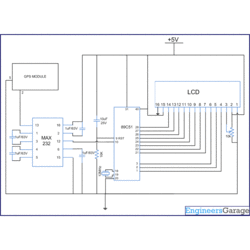# How to interface GPS with 8051 Microcontroller (AT89C51)

Posted by Unknown at 9:22 PMGPS has become an efficient tool in the field of scientific use, commerce, surveillance and tracking. This project presents a small application based on Global Positioning System. It depicts the use of GPS module/receiver to find latitude and longitude of its location. The data obtained from GPS receiver (GPGGA sentence) is processed by the microcontroller to extract its latitude and longitude values.

The GPS Module has been interfaced with
AT89C51 and the location values are displayed on a 16x2 LCD interface.

• The GPS module continuously transmits serial data (RS232 protocol) in the form of sentences according to NMEA standards. The latitude and longitude values of the location are contained in the GPGGA sentence (refer NMEA format). In this program, these values are extracted from the GPGGA sentence and are displayed on LCD.

The serial data is taken from the GPS module through
MAX232 into the SBUF register of 8051 controller (refer serial interfacing with 8051). The serial data from the GPS receiver is taken by using the Serial Interrupt of the controller. This data consists of a sequence of NMEA sentences from which GPGGA sentence is identified and processed.

The extraction of location values is done as follows. The first six bytes of the data received are compared with the pre-stored (\$GPGGA) string and if matched then only data is further accounted for; otherwise the process is repeated again. From the comma delimited GPGGA sentence, latitude and longitude positions are extracted by finding the respective comma positions and extracting the data. The latitude and longitude positions extracted are displayed on the
LCD interfaced with AT89C51.

To obtain more details (other than latitude and longitude) from the GPS receiver, GPRMS sentence can be used. Refer next article.

The circuit connections are as follows:
Receiver1 (R1) of MAX232 has been used for the serial communication. The receiver pin of GPS module is connected to R1IN (pin13) of MAX232. R1OUT (pin 12) of MAX232 is connected to RxD (P3.0) of AT89C51.

Pins 1-3 of port P1 (P1.0, P1.1 & P1.2 respectively) of AT89C51 are connected to the control pins (RS, R/W& EN) of LCD. The data pins of LCD are connected to Port P2 of the controller. The latitude and longitude positions are displayed on the LCD.

•• ```
```
/* Basic program to show latitude and longitude on LCD extracted from GPGGA statement */

#include<reg51.h>

#define port2 P2

sbit rs = P1^0;

sbit rw = P1^1;

sbit e = P1^2;

char info;

char test={"\$GPGGA"};

char comma_position;

unsigned int check=0,i;

unsigned char a;

void lcd_latitude();

void lcd_longitude();

//DELAY FUNCTION

void delay(unsigned int msec)

{

int i,j ;

for(i=0;i<msec;i++)

for(j=0;j<1275;j++);

}

// LCD COMMAND SENDING FUNCTION

void lcd_cmd(unsigned char item)

{

port2 = item;

rs= 0;

rw=0;

e=1;

delay(1);

e=0;

return;

}

// LCD DATA SENDING FUNCTION

void lcd_data(unsigned char item)

{

port2 = item;

rs= 1;

rw=0;

e=1;

delay(1);

e=0;

return;

}

// LCD STRING SENDING FUNCTION

void lcd_string(unsigned char *str)

{

int i=0;

while(str[i]!='\0')

{

lcd_data(str[i]);

i++;

delay(10);

}

return;

}

// SERIAL PORT SETTING

void serial()

{

TMOD=0x20;      //MODE=2

TH1=0xfa;     // 4800 BAUD

SCON=0x50  ;    // SERIAL MODE 1 ,8- BIT DATA ,1 STOP BIT ,1 START BIT , RECEIVING ON

TR1=1;         //TIMER START

}

void find_comma()

{

unsigned int i,count=0;

for(i=0;i<70;i++)

{

if(info[i]==',')

{

comma_position[count++]=i;

}

}

}

void compare()

{

IE=0x00;       //Interrupt disable

find_comma();   //Function to detect position of comma in the string

lcd_latitude();   //Function to show Latitude

lcd_longitude(); //Function to show Longitude

check=0;

IE=0x90;   //Interrupt enable

}

{

if(check<7)         //Condition to check the required data

{

if(info[check-1]!=test[check-1])

check=0;

}

RI=0;

}

void lcd_shape()      //Function to create shape of degree

{

lcd_cmd(64);

lcd_data(10);

lcd_data(17);

lcd_data(17);

lcd_data(10);

lcd_data(0);

lcd_data(0);

lcd_data(0);

lcd_data(0);

}

void lcd_latitude() //Function to display Latitude

{

unsigned int c2=comma_position; //Position of second comma

lcd_shape();

lcd_cmd(0x01);         // Clear LCD display

lcd_cmd(0x84);         //Move cursor to position 6 of line 1

lcd_string("LATITUDE"); //Showing Latitude

lcd_cmd(0xC0); //Beginning of second line

lcd_data(info[c2+1]);

lcd_data(info[c2+2]);

lcd_data(0); //Degree symbol

lcd_data(info[c2+3]);

lcd_data(info[c2+4]);

lcd_data(info[c2+5]);

lcd_data(info[c2+6]);

lcd_data(info[c2+7]);

lcd_data(info[c2+8]);

lcd_data(info[c2+9]);

lcd_data(0x27);          //ASCII of minute sign(')

lcd_data(info[c2+10]);

lcd_data(info[c2+11]);

delay(250);

}

void lcd_longitude()

{

unsigned int c4=comma_position;

lcd_cmd(0x01);            //Clear LCD display

lcd_cmd(0x84);            //Move cursor to position 4 of line 1

lcd_string("LONGITUDE"); //Showing Longitude

lcd_cmd(0xC0);    //Begining of second line

lcd_data(info[c4+1]);

lcd_data(info[c4+2]);

lcd_data(info[c4+3]);

lcd_data(0);

lcd_data(info[c4+4]);

lcd_data(info[c4+5]);

lcd_data(info[c4+6]);

lcd_data(info[c4+7]);

lcd_data(info[c4+8]);

lcd_data(info[c4+9]);

lcd_data(info[c4+10]);

lcd_data(0x27);               //ASCII of minute sign(')

lcd_data(info[c4+11]);

lcd_data(info[c4+12]);

delay(250);

}

void main()

{

serial();

lcd_cmd(0x38);        //2 LINE, 5X7 MATRIX

IE=0x90;

while(1)

{

if(check==69)

compare();

}

}
```

```
• AT89C51 Microcontroller
MAX232
LCD
Tags:

#### 1 comment:

1.thanks for the post
can u please mail me the details of the purpose of the variables used in the program i could not understand their use in some cases

THANKS FOR UR COMMENT ....

## Disclaimer

This blogs is an effort to club the scattered information about engineering and project titles and ideas available in the web. While every effort is made to ensure the accuracy of the information on this site, no liability is accepted for any consequences of using it. Most of the material and information are taken from other blogs and site with the help of search engines. If any posts here are hitting the copyrights of any publishers, kindly mail the details to educations360@gmail.com It will be removed immediately.

## Alexa Rank